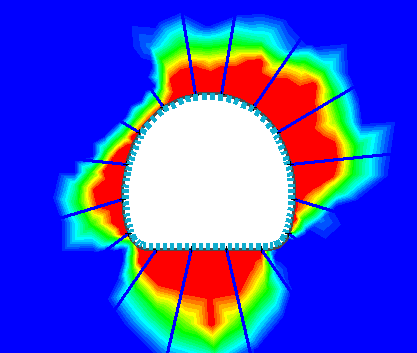Expert Modelling: Hands-on help from Rocscience Engineers. Register Here

# Yielded Elements

The Yielded Elements contouring option allows the user to contour the degree of yielding in the rock mass around excavations. The Yielded Elements option will only be available in the toolbar list in Interpret, if at least one material has been defined with Plastic material properties. If all materials are Elastic, then Yielded Elements contouring will not be available.

The Yielded Element contours are determined as follows:

1. At each node of the finite element mesh:
• the total number of elements connected to a node is determined.
• the number of yielded elements connected to a node is determined.
2. The "percent yielding" at a node, is then given by the number of yielded elements, divided by the total number of elements attached to a node. For example, if the total number of elements attached to a node is 6, and the number of yielded elements attached to the node is 2, then the "percent yielding" at that node, is 2 / 6 = 33.33 percent.
3. NOTE: for the purpose of determining the number of yielded elements at a node, an element may fail in shear only, tension only, or both shear and tension. No distinction is made between the mode of yielding, for the purpose of the yielded element contours.

4. The values of "percent yielding", obtained as described above, are then contoured like any other data type available in RS2 (e.g. Sigma 1, Strength Factor etc), as shown in the following figure.

Yielded Element contours## Tips

There are various other ways of displaying yielding in RS2 Interpret.

• The yielded elements may be displayed directly with the Show Yield option.
• The zone of yielding around an excavation, can be highlighted by displaying a Yield Line around a selected area.

The Yielded Element contours can be considered an alternative representation of yielding in the rock mass, and can be used in conjunction with these other options.